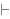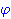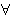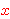New Foundations Explorer < Previous   Next > Nearby theorems Mirrors  >  Home  >  NFE Home  >  Th. List  >  hbth Unicode version

Theorem hbth 1552
 Description: No variable is (effectively) free in a theorem. This and later "hypothesis-building" lemmas, with labels starting "hb...", allow us to construct proofs of formulas of the formfrom smaller formulas of this form. These are useful for constructing hypotheses that state "is (effectively) not free in." (Contributed by NM, 5-Aug-1993.)
Hypothesis
Ref Expression
hbth.1Assertion
Ref Expression
hbthProof of Theorem hbth
StepHypRef Expression
1 hbth.1 . . 321ax-gen 1546 . 232a1i 10 1Colors of variables: wff setvar class Syntax hints:wi 4wal 1540 This theorem was proved from axioms:  ax-1 5  ax-mp 8  ax-gen 1546 This theorem is referenced by:  nfth  1553  spfalw  1672  spimehOLD  1821
 Copyright terms: Public domain W3C validator